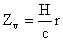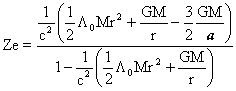(3) The computation outside the sphere

The velocity redshift is the same with in the sphereSubstitutinginto formula (12), we have(23)

where Λ0 M  and  a  is obtain from the outmost layer.

The total redshift is formula (13).

When  Zv=1 , the total redshift is  Zmax ,  and the distance  rs  corresponding to  Zmax  is just the limit of sight, that is, the limiting distance of observation.